-  Foxtable(狐表)  (http://www.foxtable.com/bbs/index.asp)
--  专家坐堂  (http://www.foxtable.com/bbs/list.asp?boardid=2)
----  [原创]通用用户权限设置图文连载（供新手参考）  (http://www.foxtable.com/bbs/dispbbs.asp?boardid=2&id=34847)

--  作者：baicaocao
--  发布时间：2013/6/17 18:09:00
--  [原创]通用用户权限设置图文连载（供新手参考）

四张表：用户登录，角色，系统信息，自动编号.

\'看了好多同学说出错，所以，我直接把项目文件上传上来。大家直接看项目文件就可以了。

admin密码为:123

 下载信息  [文件大小：   下载次数： ]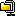点击浏览该文件:权限维护.zip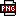此主题相关图片如下：t1.png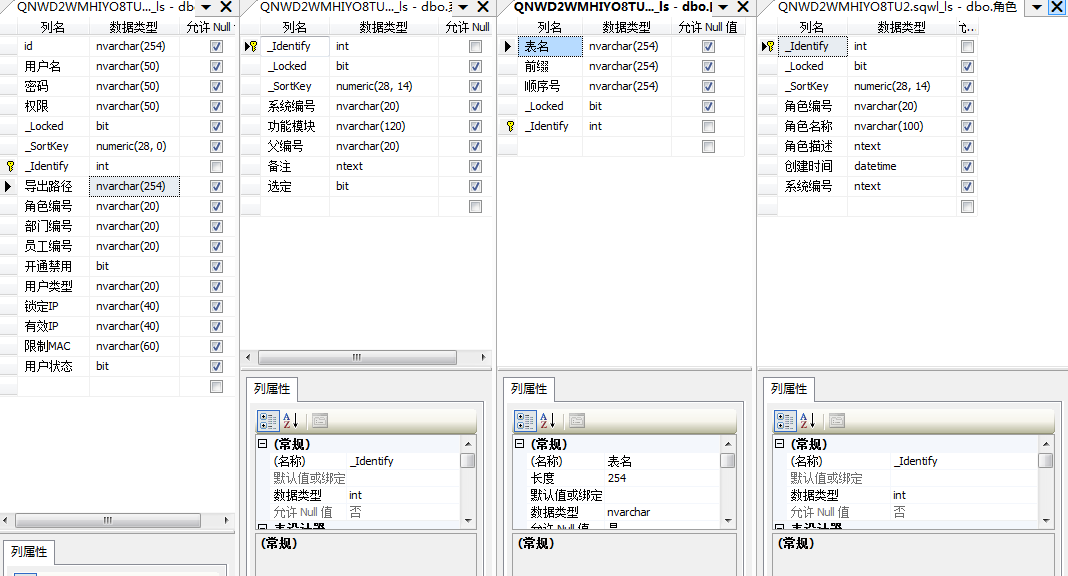此主题相关图片如下：t2.png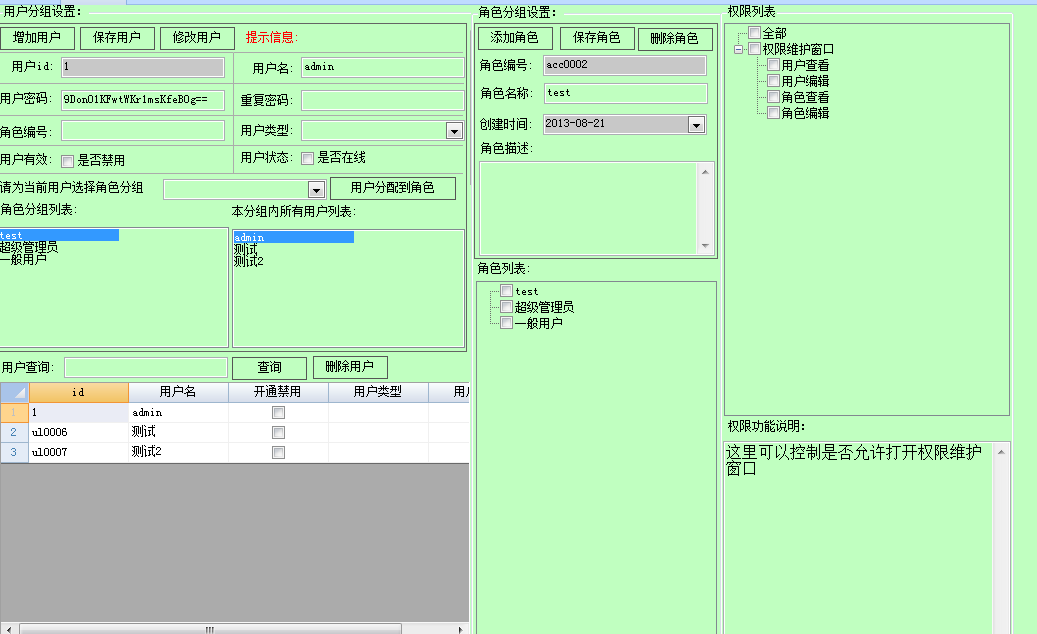此主题相关图片如下：t3.png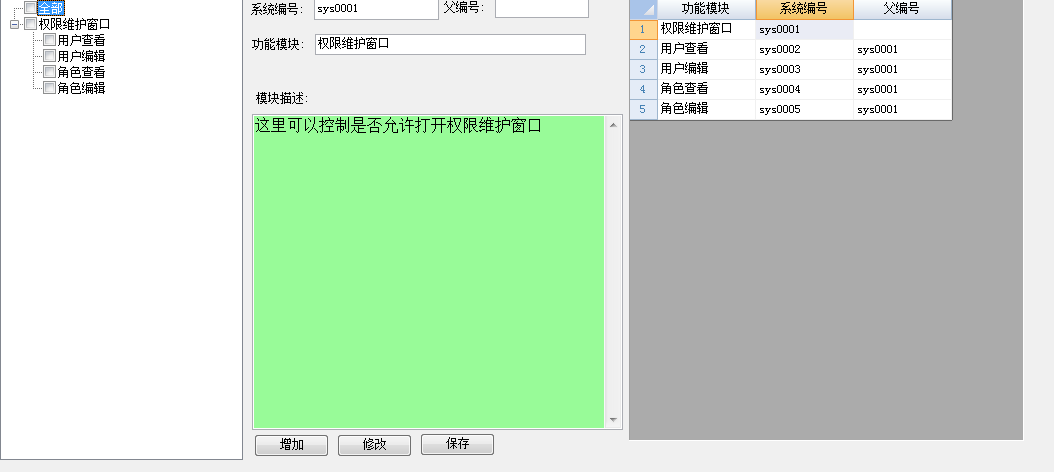1.Functions.Execute("add")函数参数2个。函数内容：

Dim nd As WinForm.TreeNode = args(0)
Dim dts As DataTable = args(1)
Dim drs As List(of DataRow)
drs = dts.Select("[父编号] = \'" & nd.Name & "\'")
For Each dr As DataRow In drs
Dim cd As Winform.TreeNode = nd.Nodes.Add(dr("系统编号"),dr("功能模块"))
Functions.Execute("Add",cd,dts)
Next

2.Functions.Execute("reflash"),参数1,函数内容：

Dim tr As WinForm.TreeView = args(0)
Dim nd As WinForm.TreeNode
Dim dts As DataTable = DataTables("系统信息")
Dim dm As DataRow
tr.StopRedraw()
tr.Nodes.Clear
tr.Nodes.Add("全部")
For Each dr As DataRow In dts.datarows
If dr.IsNull("父编号") Then
nd = tr.Nodes.Add(dr("系统编号"),dr("功能模块"))
Functions.Execute("add",nd,dts)
End If
Next
tr.ResumeRedraw()
If pw = True Then
For Each nds As WinForm.TreeNode In tr.AllNodes
nds.Checked = 权限集合.Contains(nds.Name)
Next
End If
tr.ExpandAll

3.Functions.Execute("保存用户")参数1.函数内容：

Dim frm As winform.Form = args(0)
Dim users As WinForm.TextBox = frm.Controls("TextBox2")
Dim pass As WinForm.TextBox = frm.Controls("TextBox3")
Dim apass As WinForm.TextBox = frm.Controls("TextBox10")
Dim lb As WinForm.Label = frm.Controls("Label18")
Dim dtb As DataTable = DataTables("权限维护_table1")
Dim tbs As Table = Tables("权限维护_table1")
Dim drs As List(Of DataRow)
drs = dtb.Select("用户名 =\'" & users.value & "\'")
If drs.Count >1 Then
MessageBox.Show("用户列表中用户名称重复,不能保存,请查询修改.")
Return True
End If
If tbs.Current Is Nothing Then
Else
If tbs.Current.DataRow.RowState = DataRowState.Added Then
If pass.value = "" Then
lb.text = "密码不能为空!"
Return True
Else
lb.text = "提示信息:"
End If
If pass.Value.Length <3 Then

lb.Text = "提示信息:密码应该不小于3"
Return True
Else
lb.text = "提示信息:"
End If
If pass.Value <> apass.Value Then
lb.Text = "提示信息:重复密码不一致!"
Return True
Else
lb.text = "提示信息:"
End If
Dim jm As String =EncryptText(pass.Value,"lizhen","sq#")
apass.Value = jm
tbs.Current("密码") = jm

ElseIf tbs.Current.DataRow.RowState = DataRowState.Modified Then
If Apass.Value = "" Then
tbs.Current("密码") =  tbs.Current.DataRow.OriginalValue("密码")
ElseIf Apass.value > "" Then
If apass.value <> pass.value Then
tbs.Current("密码") =  tbs.Current.DataRow.OriginalValue("密码")
lb.Text = "提示信息:重复密码不一致!"
Return True
Else
Dim jmp As String =EncryptText(pass.Value,"lizhen","sq#")
apass.Value = jmp
tbs.Current("密码") = jmp
lb.Text = "提示信息:"
End If
End If
End If
End If

\'MessageBox.Show(1)
pause = False

---------------------------------------------接下来继续说。

[此贴子已经被作者于2013-9-8 18:19:50编辑过]

--  作者：everybody
--  发布时间：2013/6/17 20:20:00
--

--  作者：zerov
--  发布时间：2013/6/17 22:46:00
--  回复：（baicaocao）[原创]通用用户权限设置图文连载（...

--  作者：bohe
--  发布时间：2013/6/18 11:45:00
--

--  作者：李睿涵
--  发布时间：2013/7/14 12:07:00
--

--  作者：baicaocao
--  发布时间：2013/8/26 22:50:00
--

4.Functions.Execute("更新角色列表").参数1.函数内容：

Dim frm As WinForm.Form = args(0)
Dim cm As WinForm.ComboBox = frm.Controls("ComboBox2")
Dim cm1 As WinForm.ComboBox = frm.Controls("ComboBox4")
Dim lt As WinForm.ListBox = frm.Controls("ListBox1")
cm.DisplayMember = "角色名称"
cm.ValueMember = "角色编号"
cm.DataSource = "角色"
cm1.DisplayMember = "角色名称"
cm1.ValueMember = "角色编号"
cm1.DataSource = "角色"
lt.DisplayMember = "角色名称"
lt.ValueMember = "角色编号"
lt.DataSource = "角色"

5.Functions.Execute("权限分配") 参数2.函数内容

If _usergroup = "超级管理员" Then
Return True
End If
\'___________超级管理员_____具备所有权限.
Functions.Execute("角色权限","[角色名称] = \'" & _usergroup & "\'")
\'_________________调用角色的权限集合__________________
Dim frm As String = args(0) \'窗口名字.
Dim vl As String = args(1) \'子功能模块.
\'MessageBox.Show(权限集合.count)
Dim tbs As DataTable = DataTables("系统信息")
Dim fl As String
fl = "[功能模块] = \'" & frm & "窗口 \' and [父编号] is null"
Dim dm As DataRow = tbs.Find(fl)
\'____________以上调用窗口名字,并查找出功能模块的系统编号_________________
If vl = "" Then
If 权限集合.Contains(dm("系统编号")) Then
Return True
End If
\'_____________判断子功能模块名称是否为空,如果为空,则继续执行_____________
Else
fl = "[父编号] = \'" & dm("系统编号") & "\' and [功能模块] =\'" & args(1) & "\'"
\'  MessageBox.Show(fl)
Dim dn As DataRow = tbs.Find(fl)
\'MessageBox.Show(dn("系统编号"))
If 权限集合.Contains(dn("系统编号")) Then
Return True
End If
\'_______________________查询出父编号之后.在查询功能编号.________________
End If

6.Functions.Execute("权限窗口检查")参数4.

Dim tn As String = args(0) \'表名
Dim lb As String = args(1) \' 提示信息名
Dim tx As String = args(2) \'提示信息内容
Dim cl As String = args(3) \'操作的列名
Dim js As Table = Tables(tn)
Dim ts As WinForm.Label = Forms("权限维护").Controls(lb)
For Each dm As DataRow In DataTables(tn).DataRows
If dm(cl) = "" Then
ts.text = tx
Return Nothing
Else
ts.text = "提示信息:"
End If
Next
js.AddNew()
Forms("权限维护").Controls("TextBox9").value = ""
Forms("权限维护").Controls("TextBox10").value = ""

7.Functions.Execute("目录树_载入")

Dim tr As WinForm.TreeView = args(0)
Dim a As String = args(1)
Dim b As String = args(2)
Dim dts As DataTable = DataTables("角色")
tr.StopRedraw
tr.Nodes.Clear
For Each ds As DataRow In dts.DataRows
tr.Nodes.Add(ds("角色编号"),ds("角色名称"))
Next
tr.ResumeRedraw
Functions.Execute("更新角色列表",Forms("权限维护"))

8.Functions.Execute("窗口加载")

Dim frm As winform.Form = args(0)
Select Case frm.Name
Case "权限维护"
Functions.Execute("reflash",frm.Controls("TreeView1"),DataTables("系统信息"))
Functions.Execute("目录树_载入",frm.Controls("TreeView2"),"角色","角色名称")
Dim 用户编辑 As WinForm.Panel = frm.Controls("用户编辑")
Dim 用户分组 As WinForm.GroupBox = frm.Controls("用户分组")
Dim 角色查看 As WinForm.GroupBox = frm.Controls("角色查看")
Dim btdel As WinForm.Button = frm.Controls("Button3")
Dim 角色编辑 As WinForm.Panel = frm.Controls("角色编辑")
btdel.Enabled = Functions.Execute("权限分配",frm.Name,"用户编辑")
用户分组.Enabled = Functions.Execute("权限分配",frm.Name,"用户查看")
用户编辑.Enabled = Functions.Execute("权限分配",frm.Name,"用户编辑")
角色查看.Enabled = Functions.Execute("权限分配",frm.Name,"角色查看")
角色查看.Enabled = Functions.Execute("权限分配",frm.Name,"角色编辑")

Return Nothing
Case ""
End Select

--------------------------------------------------接下来继续说。

--  作者：baicaocao
--  发布时间：2013/8/26 22:53:00
--

9。Functions.Execute("编码")

Dim tb As Table = args(0) \'要操作的表
Dim dr As DataRow
Dim cmd1 As new SQLCommand
Dim cmd2 As New SQLCommand
Dim Key As Integer
cmd1.ConnectionName = Lists
cmd2.ConnectionName = Lists
cmd1.CommandText = "Select Count(*) From [自动编号] Where [表名] = \'" & tb.Name & "\'"
\'If cmd1.ExecuteScalar = 0 Then \'如果编号表不存在前缀的行,那么增加一行
\'cmd1.commandtext = "Insert Into 自动编号 (前缀, 顺序号) Values(\'" & pf & "\',1)"
\'cmd1.ExecuteNonQuery
\'End If
cmd1.commandText = "Select [顺序号] From [自动编号] Where [表名]=\'" & tb.Name & "\'"
Do

Key = cmd1.ExecuteScalar() \'从后台获得顺序号
cmd2.commandText = "Update [自动编号] Set [顺序号] = " & (Key + 1) & " Where [表名] = \'" & tb.Name & "\'"
If cmd2.ExecuteNonQuery() > 0 Then \'更新顺序号
Exit Do \'更新成功则退出循环
End If
Loop
\'________________前缀提取_______________
cmd1.commandText = "Select [前缀] From [自动编号] Where [表名]=\'" & tb.Name & "\'"
Return cmd1.ExecuteScalar() & Format(Key,"0000")

10.Functions.Execute("角色操作")

Dim 角色名称 As String = args(0)
Dim 系统编号 As String = args(1)
Dim px As Boolean = args(2)
Dim dm As DataRow
Dim Arys() As String
Dim lv As String
Functions.Execute("角色权限","角色编号 = \'" & 角色名称 & "\'")
Select Case px
Case True
If 权限集合.Contains(系统编号) Then
Else
权限集合.Add(系统编号)
End If
Case False
If 权限集合.Contains(系统编号) Then
权限集合.Remove(系统编号)
End If
End Select
Arys = 权限集合.ToArray()
lv = String.Join(",",Arys)
dm= DataTables("角色").Find("角色编号 = \'" & 角色名称 & "\'")
dm("系统编号") = lv

11.Functions.Execute("角色权限")

Dim fl As String = args(0)
Dim dm As DataRow = DataTables("角色").Find(fl)
Dim m As String = dm("系统编号")
Dim k() As String = m.Split(",")
For i As Integer = 权限集合.count -1 To 0 Step -1
权限集合.RemoveAt(i)
Next
\'清除权限集合中的数据.
\'MessageBox.Show(k.Length)
\'If k.Length > 1 Then
权限集合.AddRange(k)
\'End If
\'Return 权限集合

12.Functions.Execute("角色目录树复选")

Dim frm As WinForm.Form = args(0)
Dim tr As WinForm.TreeView = frm.Controls("TreeView2")
Dim 角色名称 As WinForm.Label = frm.Controls("角色名称")
Select Case tr.SelectedNode.Checked
Case True
For Each nds As WinForm.TreeNode In tr.AllNodes
\'dm=dts.Find("系统编号 = \'" & nds.Name & "\'")
If nds.Name <> tr.SelectedNode.Name Then
nds.Checked = False
End If
Next
\'MessageBox.Show(3)
角色名称.Text = tr.SelectedNode.Name
\'  MessageBox.Show(tr.SelectedNode.Name)
Functions.Execute("角色权限","[角色编号] = \'" & tr.SelectedNode.Name & "\'")
pw = True
Functions.Execute("reflash",frm.Controls("TreeView1"))
pw = False
Case False
End Select

\'-------------------------------所有函数已经列举完毕。下面继续。

--  作者：有点甜
--  发布时间：2013/8/26 23:00:00
--
嘻嘻嘻，这个，真不错，建议狐表要集成权限管理的帮助，这块确实不完整。
--  作者：baicaocao
--  发布时间：2013/8/26 23:08:00
--

AfterOpenProject 事件。

server = "server"
Lists = "list"

\'------------

Public pause As Boolean
Public pw As Boolean \'权限状态
Public _UserName As String \'用户名
Public dt As DataTable \'临时表定义.
Public server As String  \'外部数据源
Public Lists As String  \'外部数据源
Public 权限集合 As New List(Of String)
‘------------------------下面是窗口

1.编码窗口: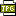此主题相关图片如下：5.jpg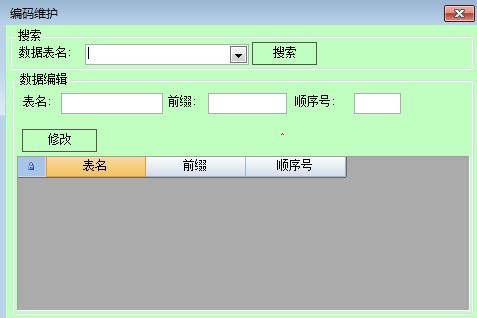这个文件是开发板窗口。

[此贴子已经被作者于2013-8-27 7:37:22编辑过]

--  作者：swd84926741
--  发布时间：2013/8/27 9:15:00
--